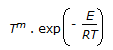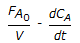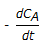# Chemical Engineering - Chemical Reaction Engineering

### Exercise :: Chemical Reaction Engineering - Section 7

1.

The minimum energy required to allow a chemical reaction to proceed is termed as the 'threshold energy '. Chemical reaction with low activation energy are

 A. always irreversible. B. insensitive to temperature changes. C. mostly irreversible. D. highly temperature sensitive.

Explanation:

No answer description available for this question. Let us discuss.

2.

If Thiele modulus is __________ , then the pore diffusion resistance in a catalyst may be considered as negligible.

 A. 0 B. ∞ C. < 0.5 D. > 0.5

Explanation:

No answer description available for this question. Let us discuss.

3.

From collison theory, the reaction rate constant is proportional to

 A.B.C. T D.Explanation:

No answer description available for this question. Let us discuss.

4.

For a mixed flow reactor operating at steady state, the rate of reaction is given by

 A.B.C.D.Explanation:

No answer description available for this question. Let us discuss.

5.

Catalytic action in a catalytic chemical reaction follows from the ability of catalyst to change the

 A. activation energy B. equilibrium constant C. heat of reaction D. none of these